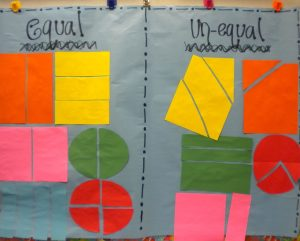# Fractions Part I: Basics KG-2nd grade

by C. Elkins, OK Math and Reading LadyThis is the first post of several I will devote to fractions, starting with basic understanding in first grade and moving up toward operations with fractions in 5th and 6th grade. I would love to hear from you about your students successes and/or difficulties with fractions so I can be sure to address this topic to meet your needs. Free resources below.

What is a fraction?  A fraction represents a part of a whole. It consists of a numerator (which tells how many parts we are describing) and a denominator (how many parts the whole is divided into).

Some basics:

• Fractional parts must be equal. This is a concept introduced in first grade. (See some lesson plan ideas below.)From Pinterest

• The larger the denominator, the smaller the parts AND the smaller the denominator, the larger the parts — when comparing identical sized objects. You can’t compare 1/4 of a cookie with 1/4 of a cake. This is one of the hardest concepts to grasp – so lots of hands-on experience is needed.
• Helpful manipulatives to use with fractions: pattern blocks, color tiles, Cuisenaire rods, fraction circles, fraction strips, fraction bars, graham crackers.
• Be careful about always referring to fractions as “the shaded part.” While this might be true with pictures on worksheets, fractions can be described in these ways also: What fraction of the students are boys? What fraction of the pizza was eaten? What fraction of the candy bar is left? What fraction would belong here on the number line?
• Lines do not necessarily define the fractional part. On the picture shown, the left shows 1/4 shaded. The right also shows 1/4 shaded, but students are likely to say 1/3. Why? Because they count the parts shown (3) and the shaded parts (1) and put that together as a fraction. A way to show this is still 1/4 is to show that the shaded part will fit into the whole shape 4 times.• A unit fraction: This is a fraction with 1 as the numerator (1/4, 1/8, etc.). It is one unit of the whole.
• A fraction that is one unit away from a whole has a numerator one less than the denominator. Examples: 2/3, 3/4, 7/8, 11/12, etc. This is helpful to conceptualize when comparing fractions.
• When reading a fraction number line, compare it to a bar model. Then it is easier to see it is the spaces are the focus, not the tick marks.